SocNetV Manual

Social Network Visualizer (SocNetV) is a cross-platform, user-friendly free software application for social network analysis and visualization. Social Networks are created or imported from files and are drawn as graphs, where vertices depict actors or agents and edges represent their ties.

This is the Manual of SocNetV which is divided into the following sections:

If you are interested in the algorithms behind Social Network Visualizer, you can browse the annotated version of the source code. If you are a C++/Qt developer, you can help our project with new features and bugfixes. Feel free to checkout our source code development tree.

# Introduction

## What is Social Network Analysis?

A Social Network is the social structure which facilitates communication between a group of actors (individuals or organizations) that are related somehow (i.e. by common interests, shared values, financial exchanges, friendship, dislike, etc).

For instance, your friends and you form a social network. But, social networks operate on many more levels, from family relations and disease spreading up to the level of company strategies, social movements or even nations.

Furthermore, research in many scientific areas has shown that social networks are important when we study the way problems are solved, diseases are spread, organizations are run, and the degree to which individuals succeed in achieving their goals.

Social Network Analysis (SNA) is a beautiful blend of Sociology and Mathematics, composed of various interdisciplinary techniques for the study of such social networks.

SNA researchers conceptualize social relationships in terms of nodes and edges (links) in mathematical graphs.

Nodes represent the individual actors within the networks, while edges visualize the relationships between those actors.

The result is graph-based structures which are often very complex to understand and analyze. This is where applications like SocNetV are needed.

## What is SocNetV?

Social Network Visualizer (SocNetV) is an open-source project to build a flexible and user-friendly, cross-platform tool for social network analysis and visualization, targeting primarily the social researcher. It is developed in C++ and Qt, an open-source development toolkit and runs on Linux, OS X and Windows.

SocNetV offers an easy to use Graphical User Interface (see User Interface overview), which lets you construct social networks with a few clicks on a virtual canvas or load networks of various formats (GraphML, GraphViz, Adjacency, EdgeList, Pajek, UCINET, GML, etc, see more in Supported Formats) and modify them to suit your needs.

Random networks can be created using various random network generation models (Barabási–Albert Scale-Free, Erdős–Rényi, Watts-Strogatz Small-World, d-regular, ring lattice, etc). Read more about them in section Random network creation.

The application computes standard graph theory and network cohesion metrics, such as density, diameter, geodesic distances (shortest path lengths), clustering coefficient (see Coefficient), walks, connectedness, eccentricity, etc. Read details in: Cohesion measures.

It also offers structural statistics, such as node and network centrality and prestige metrics, i.e. betweenness, eigenvector and closeness centrality, proximity and pagerank prestige. Read more in: Centralities and Prestige.

Fast algorithms for community detection, such as triad census, clique census, etc are included. Read more in: Community detection.

With SocNetV you can also perform structural equivalence analysis, using hierarchical clustering, actor similarities and tie profile dissimilarities, Pearson coefficients, etc. See more in Structural Equivalence methods.

For meaningful visualization of a social network, SocNetV supports various layout algorithms and models. For example, you can embed a layout model (radial, level, nodal sizes and color) based on a prominence index (i.e. Degree Centrality score). Or you can choose one of the force-directed placement algorithms (i.e. Kamada-Kawai Spring Embedder, Fruchterman-Reingold, etc). See more in Visualization and layout algorithms.SocNetV loaded with a 65-actor network visualized in FDP layout. Actors colors and size denote their Eigenvector centrality.
Warning
{ SocNetV is a work in progress. See Known-bugs and bug reporting. }

The program is Free Software, licensed under the GNU General Public License 3 (GPL3). You can copy it as many times as you wish, or even modify it provided you keep the same license.

The documentation is also Free, licensed under the Free Documentation License (FDL).

## Installation

All three major Operating Systems are supported: Windows, macOS and Linux distributions.

You can download an installer for Windows, or a disk image for MacOS or install binary packages for Linux.

If you prefer to compile the application from scratch, source code archives are also available. Read detailed instructions below.

#### Windows

To install and run SocNetV in Windows, download the latest SocNetV installer for Windows from our Downloads page. It will be a file with a name like this: SocNetV-3.1-3fa9cf7f-windows-installer.exe

Double-click on that executable to start the installation.

Note: You might see a Windows pop up about unknown software origin/publisher. Please ignore it and proceed, as we do not sign our released packages with any code signing certificates (which are not free by the way).

Click Next and Accept the License (GPL) to install the program.

The program will be installed in the usual Windows Program Files directory and a new Start Menu shortcut will be created.

Note: Windows Defender might alert you about a threat in SocNetV windows installer. This is a false positive as the installer is automatically created in Appveyor CI and checked with VirusTotal. Our installer is 100% safe. For your peace of mind, scan the installed files with Defender to verify it for yourself.

#### MacOS

If you are a Mac user, download and install SocNetV from our official disk image (.dmg file).

From our Downloads page, download the MacOS .dmg file, i.e SocNetV-v3.1-macos.dmg.

The application is now installed. You can find it in your Applications and run it by double-clicking on its icon.

Note: The first time you will run the SocNetV app or the installer, macOS may tell you that it cannot verify the software developer/publisher. That's because we do not sign our released packages with any code signing certificates (which are not free, by the way). Please press Cancel, not Move to Bin! Then, right-click again on the app (while pressing the Ctrl key) and select Open to run the application normally. To install SocNetV, just drag the app icon to your Applications folder.

Alternatively, Szabolcs Horvát has contributed a SocNetV port to MacPorts. It can be installed with the command:

port install socnetv.

#### Linux

This is an self-contained image which allows you to run SocNetV without even installing it.

chmod +x SocNetV-v3.1-x86_64.AppImage

Then double-click on it to run the program.

Please note that SocNetV is also available in the repositories of most Linux distributions. However the package there is not always the most recent version. We urge you to use the AppImage of the latest version available from our website instead.

#### Debian

For Debian GNU/Linux, a version of SocNetV can be found in the official Debian repositories. However, that version is not always the latest and greatest. We suggest to download the Linux AppImage from our Downloads page as described above.

Alternatively, in Debian and Ubuntu, you can try to add our repository and install SocNetV with these commands:

sudo apt-get update
sudo apt-get install socnetv

This repository is signed with 61AE869C37A4FCC5A73FD02EE088941209CFE071 OpenPGP key.

To add our PPA's key to your system, open a terminal and enter this command:

sudo apt-key adv --recv-keys --keyserver keyserver.ubuntu.com 61AE869C37A4FCC5A73FD02EE088941209CFE071

#### openSUSE

For installation in openSUSE, you can add our our openSUSE repository and install the program:

sudo zypper in socnetv

#### Fedora

Fedora and EPEL users can install the latest SocNetV version from the official repositories.

For instance, to install SocNetV in Fedora 38, use the command:

sudo yum install socnetv

#### Arch Linux (AUR)

Tom Tryfonidis maintains a SocNetV package in AUR. You can download and compile it from https://aur.archlinux.org/packages?O=0&K=socnetv.

#### Source Code Compilation

To compile SocNetV from source code, you need the Qt6 development libraries installed. Most Linux distributions offer Qt6 via their package manager.

You will need the following packages, at least:

openSUSE: qt6-base libqt6-qtbase-devel qt6-charts qt6-tools qt6-qt5compat

Fedora: qt6-qtbase qt6-qtbase-devel qt6-qtcharts-devel qt6-qttools qt6-qt5compat

Debian/Ubuntu: qt6-default libqt6charts6-dev qt6-5compat-dev

After you install Qt6, download the SocNetV source code archive from our Downloads page, uncompress it, enter the new directory, and compile with the following commands.

unzip app-master.zip
cd app-master
qmake
make
sudo make install
Note
We suggest to avoid compilation and use the installers and packages we offer (see above).

#### Development version

If you want to test the latest/current development version of SocNetV, check it out using this command (you need the git package installed in your computer):

git clone -b develop --single-branch https://github.com/socnetv/app.git socnetv

Unzip, enter the new directory, then type in the commands:

qmake
make
socnetv
Note
The development version is not always stable.

## Execution Options

If you run SocNetV from the command prompt, there are three (at the moment) options: --version | -V Displays the version of the program.

--help | -H Displays a short help message.

file.net The name of the file you want to open.

# User Interface overview

SocNetV has a simple Graphical User Interface (GUI) composed of:

The main part of the application window is occupied by the sidebar panels (left-right) and the virtual "canvas" where the network appears.

You interact with the nodes and edges of the network with your mouse.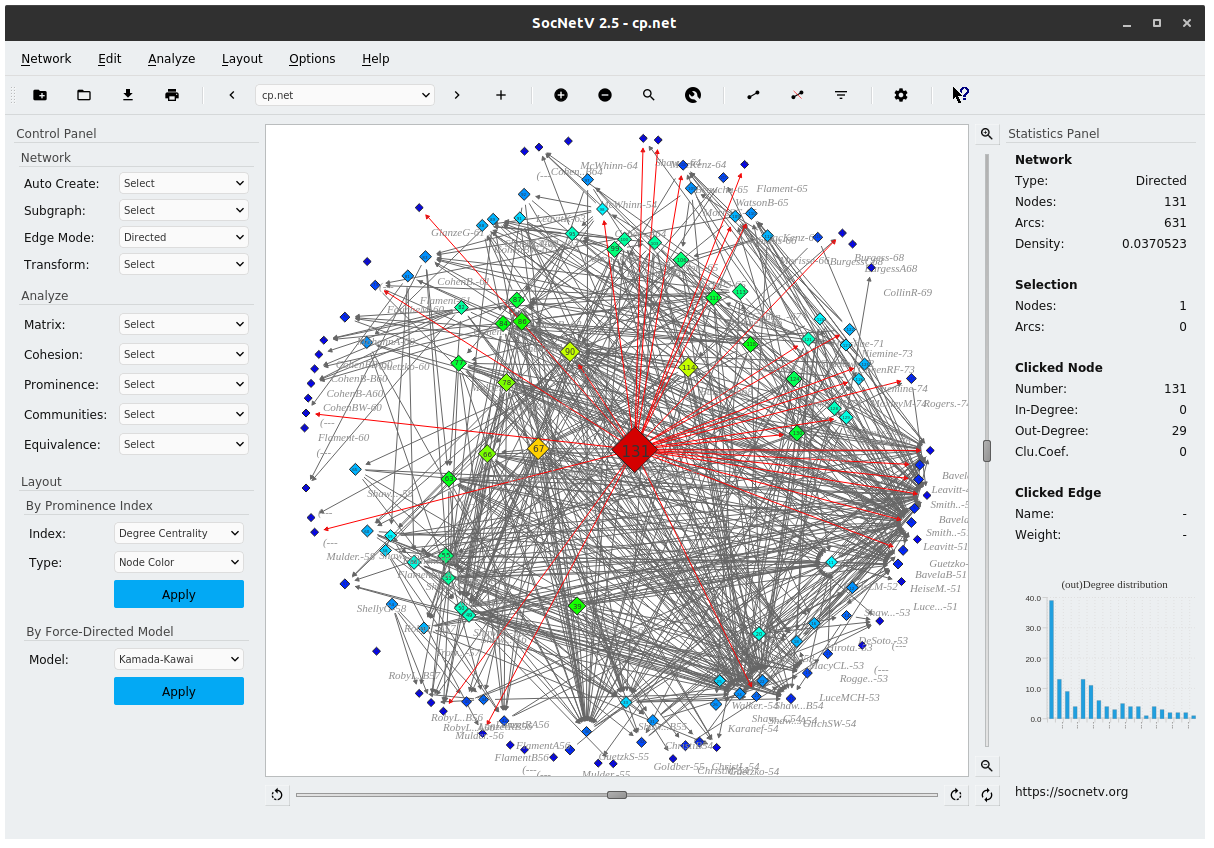Example of SocNetV Main Window

At the top of the window, there is the menu bar, filled with commands and options, organized in 6 menus:

• Network: Actions to load and save a network, export it, create random nets, operate the web crawler etc.
• Edit: Options to add/remove nodes and edges, change colors, filter edges/isolate nodes, etc.
• Analyze: This menu holds all the essential commands to analyze the active network: Matrix analysis, Cohesion analysis, Prominence (centralitiy and prestige) measures, Communities and subgroups, structural equivalence methods etc).
• Layout: Commands to apply layout models. There are two main groups of models. The first group models the network according to a prominence index (i.e. Betweenness Centrality) and a selected layout type (radial, level, nodesize or nodecolor). The second group uses force-directed placement algorithms for layout, such as Kamada-Kawai.
• Options:Allows you to show/hide edges, edge arrows, turn on/off antialiasing, etc.
• Help

## The toolbar

Below the menu, the toolbar lets you carry out a range of actions with a single click.

You can create a new net, load a network file, save current network, and print the network.

Also you can switch between relations, add new relations and display help messages for the menu options.

In the toolbar, there are buttons to edit the nodes (add/remove/find/properties), the edges (add/remove/filter) and open the application Settings.

## The Panels

The panel on the left of the window is the Control Panel. The panel on the right is the Statistics Panel.

#### Control Panel

The Control Panel is comprised of 3 groups of actions/commands:

• Network
• Analyze
• Layout

Most of these actions are the same with the ones in the application's main menu. They appear in the Control Panel for ease of use.

In the Network group, there are 4 sub-menus:

• Auto-create to automatically create random networks,
• Subgraphs to create a network from selected nodes,
• Edge mode to switch the Edge mode (directed/undirected)
• Transform, to transform the netowk, i.e. symmetrize edges.

The Analyze group offers all analysis measures and methods supported by SocNetV, categorized in five sub-menus:

• Matrix: Commands to compute and display the adjacency matrix and other matrices based on the adjacency, such as Laplacian matrix, Degree Matrix Cocitation Matrix. You can also plot the adjacency matrix.
• Cohesion: Measures related to the cohesion of the network. Reciprocity, Symmetry, Geodesic Distances & Average Distance, Eccentricity, Network Diameter as well as Connectedness, Clustering Coefficient, Walks and Reachability.
• Prominence: Methods to measure how prominent (important) each actor (node) is inside the network. Contains all Centrality and Prestige measures: Degree Centrality, Closeness Centrality, Betweenness Centrality, Stress Centrality, Eccentricity Centrality, Power Centrality, Information Centrality, Eigenvector Centrality, Degree Prestige, Pagerank Prestige, and Proximity Prestige.
• Communities: Compute cliques, triad census, etc.
• Equivalence: The structural equivalence methods that SocNetV supports, such as Hierarchical Cluster Analysis, Tie profile dissimilarities, etc.

When you select an option, SocNetV computes what you asked and displays the report (in HTML format) in a new browser window.

Note
All reports are automatically saved in a directory called "socnetv-data" in your HOME folder with a different timestamp. You can save the report from the browser window to another directory if you wish.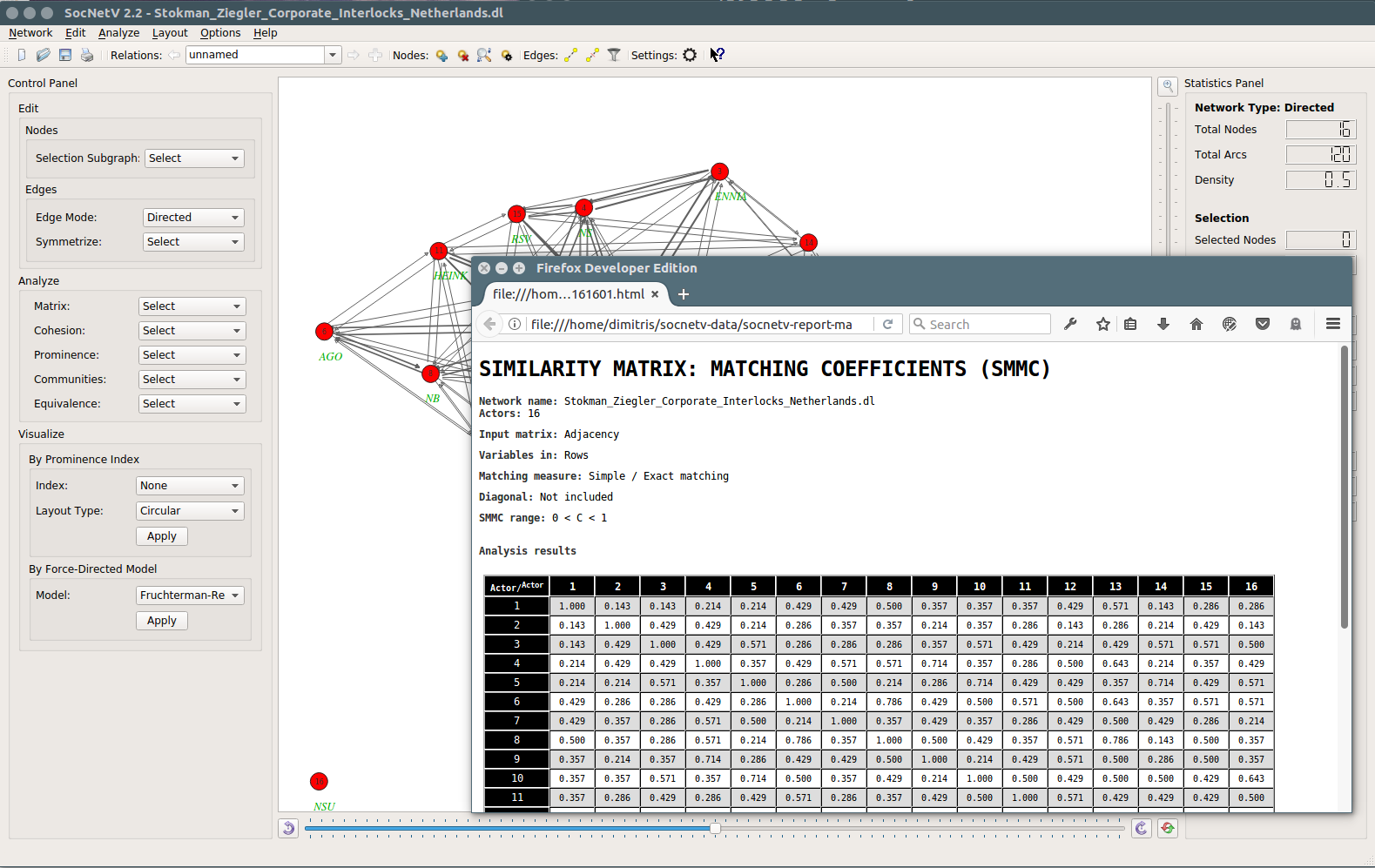Example of SocNetV report (in HTML): Pearson Coefficients

In the Layout group, you will find options to embed visualization layouts to the current network.

With one click, SocNetV can visualize the network in some intuitive ways. At the moment two layout methods are supported:

• By prominence index. Here you can select a prominence metric (i.e. Betweenness) and a layout type (i.e. circular). Hit "Apply" and voila!
• By Force Directed Placement (FDP), such as the Kamada-Kawai model.

#### Statistics Panel

The Statistics Panel shows basic information about the network (nodes, edges, density) as well as information about the selected nodes and/or the clicked node or edge.

At the top of the Panel there is a Network Type label, which indicates whether your data is directed or undirected.

Below the application displays statistics for the active network (i.e. node and edge counts, density, counts of inLinked/outLinked nodes, etc). Furthermore, under "Active Node" there are statistics which refer to the last clicked node (notably its number, in-Degree, out-Degree and Clustering Coefficient).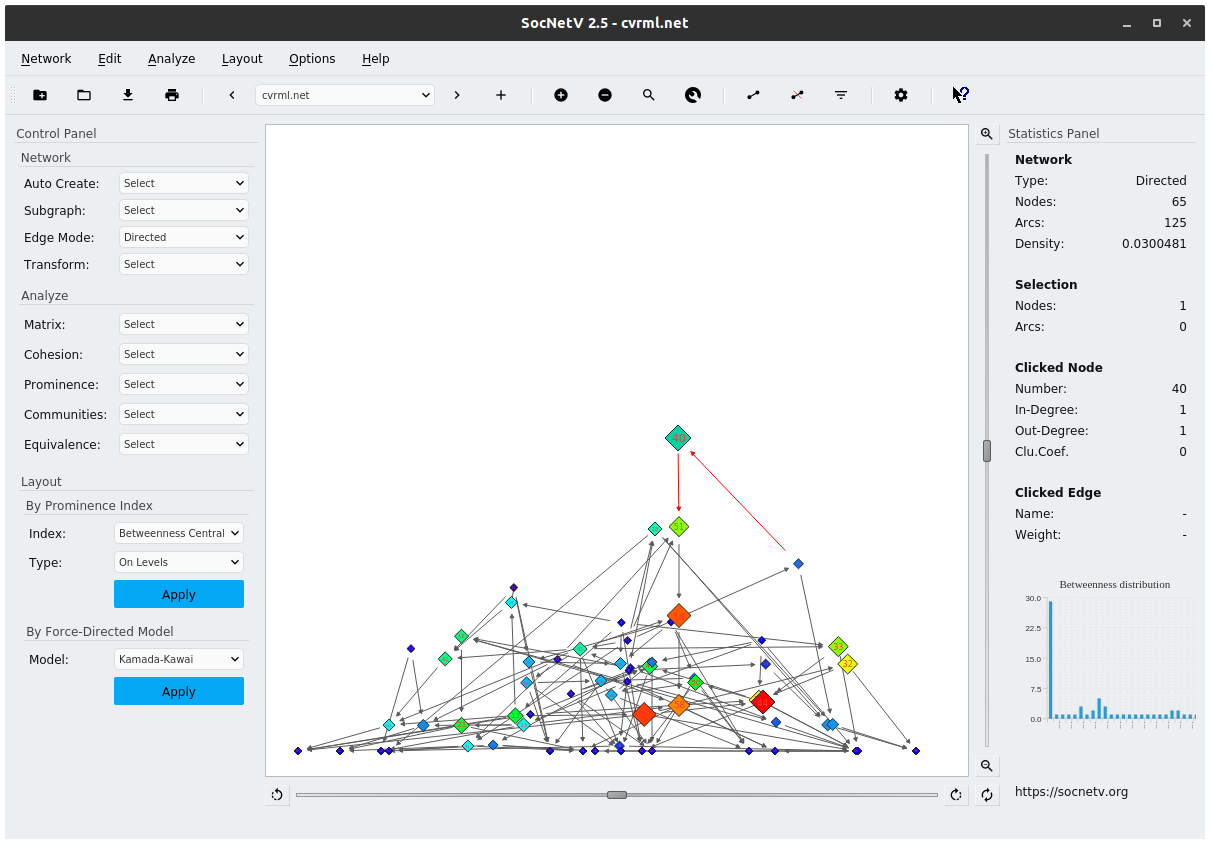Main Window

## The canvas

The canvas is the main area of interaction. You can:

• point and click on a node/edge to select it, left-click to open context menu
• double-click on empty space to add a node,
• middle-click on a node to add a directed edge from it.

The initial background color is set to "white", but you can changed it from the Edit -> Colors menu.

Below, we describe how to work with SocNetV.

## Working with SocNetV

#### Network creation

To start working with SocNetV you need network data, i.e. a graph of nodes (vertices) and links (edges).

SocNetV enables you to create networks by point and clicking on the canvas or load them from files.

There are multiple ways to create or edit nodes and links in SocNetV:

• from the left panels buttons, or
• by right/left/middle/double-clicking on the canvas.

#### Creating and handling nodes

To create a new node, you can double-click on the canvas, click on the "Add node" toolbar button or press CTRL+.

You can move a node by left-clicking on it and dragging it with mouse. When you click on a node or drag it, SocNetV highlights all its adjacent edges.

Right-click on a node to display a context menu with options to delete it, add edge, change node properties (see below). To change the color, size or label of a node, right-click on it and in the context menu select Node Properties.

All nodes by default are tagged by their node number. If you want to display the labels as well, enable the option in the menu Options -> Node -> Display Labels.

Warning
In large networks, it is sometimes difficult to locate a specific node. In such cases, you can press CTRL+F to find a desired node (by node number or label). SocNetV will highlight that node for you. Press Ctrl+F again to undo this.

When you right-click on a node, a context menu appears. From there you can remove the node, add an edge or select Node Properties to change its color, label, size, shape, etc. A similar menu appears when you right click on an edge.Node Properties dialog

From the Node Properties dialog, you can enter a node label, change the node size (and "value" in future versions), edit the node color and select a proper node shape. SocNetV supports many kinds of node shapes, i.e rectangles, diamond, ellipse, circle, etc. Click OK and all your changes will be done in one step. For instance clicking on the red color button will bring up a nice Colors dialog where you can select every possible color.

Group select

If you want to select more than one node, press and hold down the left mouse button on the canvas. By moving your mouse, a rectangle will be drawed. All nodes inside this rectangle will be selected the moment you release the mouse button. You can also edit multiple selected nodes at once. Left-click on the canvas and drag to make a selection area. Then right click on one of the selected nodes to open the Node Properties dialog for all of them. Any change you make will be applied to all selected nodes.

Warning
In networks with thousands of edges, the group selection process can be slow...

#### Creating and editing edges

There are lots of ways to create edges in SocNetV.

For instance, you can do it by middle-clicking or double-clicking on a source node and then middle-clicking or double-clicking again on a target node.

By default, all new links created this way have a unit weight (w=1). You can change the weight of an edge later by right-clicking on it and selecting "Change Weight" from the menu which appears.

Note
By default, edges are drawn so that their line width is analogous to their weight. The formula used is $$width = 1 + \log(1 + \log |weight|)$$

You can also create edges by right-clicking on any node (thus selected as source), and selecting "Create Edge". In the new dialog, just enter the target node number and the desired edge weight.

Finally, you can click on the "Add edge" button from the left Control Panel. In that case, you will be asked for both the source and the target node numbers (and the edge weight).

If you prefer using the keyboard, you can also create new edges using the keyboard shortcut CTRL+/. You will be asked for source and target nodes and their weight.

Note
When you create the first edge of a network, you will be asked to label the current Relations relationship.

Edge Creation Example:

Say you created two nodes, numbered 1 and 2, on the canvas. To create a new directed edge from node 1 to node 2, middle click on node 1 (the mouse pointer will become a hand) and afterwards middle-click on node 2. A new line will be drawn instantly. If you want an undirected edge (edge) repeat the process from node 2 to node 1.

Remember, each edge you create this way has the default weight 1 and black color.

Right-click on an edge to display a context menu with options to delete it, change weight, color etc.

Note
When you click on an edge, SocNetV highlights the source and target nodes for your convenience. Click again the edge to undo this.

## Relations

The first time you create an edge in your network, the application asks you to enter a name (or label) for the new relation between actors/nodes.

A relation is a collection of ties of a specific kind between the network actors.

For instance, you might want to label a relation "friendship" if the edges between nodes refer to the set of friendships between pairs of actors.

SocNetV supports multi-relational networks, that is networks with ties of different kind between actors.

You can add more relations to your network by pressing the + button in the toolbar.

You can switch between relations by clicking the previous and next arrow buttons in the toolbar.

Note
While modifying a multi-relational network, you can add more nodes but you may not remove a node from the network.
Warning
Each time you save a network, SocNetV saves the active relation only. You can save another relation by moving to it and then save again to another file.

## Basic functions in Network Menu

If you have network data saved in a supported format, i.e. GraphML (see Supported Formats [Formats], you can easily load that file in SocNetV.

For instance, you might have another program (for example a simulation) creating network data which you want to visualize.

From the app menu select File > Load. A file selection dialog will appear where you navigate to the desired folder and select the appropriate network file.

This menu option is more suitable for loading a network file in GraphML format (.graphml), which is the default format of SocNetV. Nevertheless, if you select a file of a different but supported format (GML, Pajek, etc), SocNetV will attempt to load it.

If you have trouble loading a network data file, and its format is supported (i.e. Pajek, UCINET, GraphViz, etc), please use the options in the Import sub menu. They are more safe.

Once you select a file, SocNetV will first display it in a File Previewer window. This allows you to select -if you need to- a specific codepage for that file. You might need this feature due to the different codepages used by the Windows, Linux and Mac operating systems. For example, say you use SocNetV in Mac OS X and you want to open a Windows network file containing non-Latin characters (such as é or some curillic "кЧДЛХКЮ"). To properly open that file you need to select its original codepage, i.e. KOI8-R. That's what File Previewer allows you to do.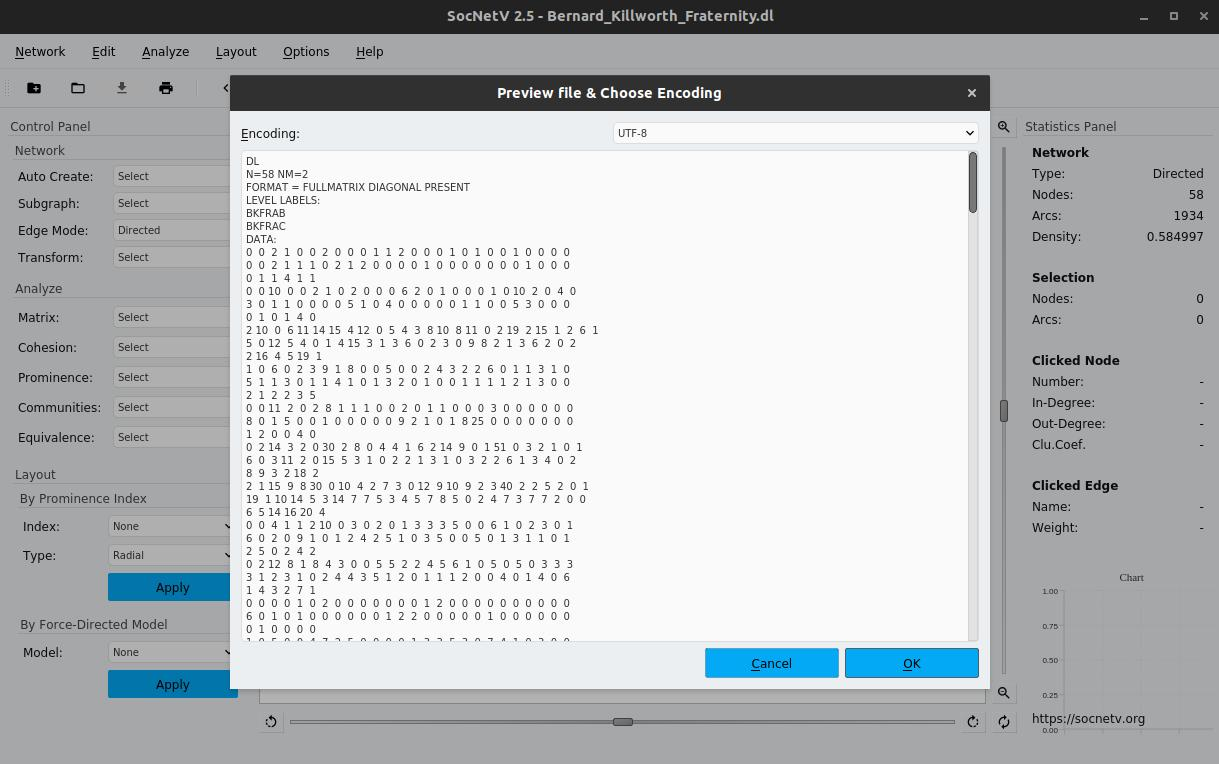In the File Previewer dialog you can select a specific codepage for the opened file -- see upper right corner

Of course, if the file has been created in the same OS as yours or by SocNetV you do not need to select another codepage, just press OK to load the network in the canvas.

Note
The default codepage for loading files is UTF-8. Select another codepage only if you know that the file comes from Windows or has non-latin characters.
Linux and Mac users should always use UTF-8, except when they try to load files saved in Windows computers.
Windows users should probably use Windows-1253, except when they want to load files saved in Mac/Linux or files containing non-latin chars (i.e. Russian).
Russian Windows users should probably use KOI8-R encoding, except when reading files saved from SocNetV (UTF-8)

#### Saving the network

To save the active network, just press Ctrl+S or click on the menu entry File > Save. By default, it will be saved in GraphML format.

If you like, you can export it to another supported format (menu Network > Export To). Note that some Supported Formats [Formats] are supported only for loading - not for saving.

Note
Each time you save a network, SocNetV saves the active relation.
By default, UTF-8 is used for writing files. This means SocNetV by default uses UTF-8 codec for all output textstreams, such as network files.

#### View or plot the adjacency matrix

The adjacency matrix of a network is a matrix where each element a(i,j) is equal to the weight of the edge from node i to node j.

If the nodes are not connected, then a(i,j)=0.

To view the adjacency matrix of a network, press F6.

By default, SocNetV displays the adjacency matrix as integer-valued only (although we do allow float weights).

You can optionally plot the adjacency matrix. Press Shift+F6 to see the plot.

#### Use a known data-set

SocNetV can recreate known data sets. See Recreating famous data-sets.

#### Random network creation

SocNetV can create a random network for you based on a selected model. See Network Generation.

#### Web Crawler

SocNetV includes a simple web crawler. See Networks of webpages using the Web Crawler.

#### Printing and Exporting

To print the active network directly to your printer, press Ctrl+P.

Keep in mind, that SocNetV follows the "what you see is what you print" principle:

we print what is viewable in the canvas, i.e. if you zoom-in to a network, the application will only print that specific network portion. So, you might need to zoom-out enough so that the whole network is viewable and therefore printable.

Except printing, you can export your work into raster (BMP and PNG) images, as well as PDF documents. PDF documents are vector-based, and therefore offer the best quality. Again, keep in mind the rule "what you see is what you print".

# Network Generation

This page has information about the network generation routines and capabilities of SocNetV. The application can either recreate famous social network analysis data sets (i.e. Knocke: Bureacracies Network) or generate random networks using a graph theory model such as the Barabási–Albert for scale-free networks and the Watts-Strogatz model for small-world nets.

SocNetV can also create "networks" of linked webpages using the built-in Web Crawler. The Crawler maps hypertext links between webpages starting from a given webpage/website.

## Recreating famous data-sets

SocNetV can recreate one of the following known social network data-sets:

• Krackhardt: High-tech managers (advice, friendship and reports to relationships), 24 actors
• Zachary: Karate Club (simple & weighted ties), 34 actors
• Bernard: Killworth Fraternity (multirelational), 58 actors
• Galaskiewicz: CEOs and clubs (affiliation data)
• Freeman's EIES networks (multirelational, 32 actors)
• Freeman: EIES network, at time-1, 48 actors
• Freeman: EIES network, at time-2, 48 actors
• Freeman: EIES network, number of messages, 48 actors
• Freeman: The 34 possible graphs with N=5 (as multirelational), 5 actors
• Mexican Power Network in the 1940s (list format)
• Knoke: Bureaucracies Network (information and money relation), 10 actors
• Stephenson and Zelen (1989): Network of 40 AIDS patiens (sex relationship)
• Stephenson and Zelen (1989): Information Centrality test dataset, 5 actors
• Stokman-Ziegler: Corporate Interlocks in Netherlands, 16 actors
• Stokman-Ziegler: Corporate Interlocks in West Germany, 15 actors
• Wasserman and Faust: star, circle and line graphs of 7 actors (multirelational)
• Wasserman and Faust: Countries Trade (basic manufactured goods), 24 actors
• Petersen graph: a non-planar, undirected graph with 10 vertices and 15 edges
• Campnet dataset: a network of 18 actors, Borgatti (1992)
• Herschel graph: The smallest nonhamiltonian polyhedral graph with 11 nodes and 18 edges

From File menu select "Create known data set" or press F7.

A dialog will appear where you select one of the data-sets above.

Press OK and the network will be displayed in the canvas.

## Random network creation

SocNetV can create a random network for you. At the moment, it can create the following types of random networks: Scale-free, Small world, Erdos-Renyi, Ring lattices, and d-regular networks.

#### Scale-free (S-F) networks

A scale-free network is a network whose degree distribution follows a power law. That is, actors with a degree that greatly exceeds the average are relatively common. These highest-degree nodes are often called "hubs" and have the so-called cumulative advantage: A node with many in-links will attract more in-links than a regular node.

For large values of degree $$d$$, the fraction $$P(d)$$ of actors in a scale-free network having $$d$$ ties to other actors is

$P(k) \text {~} k^{−γ}$

where $$γ$$ is a parameter whose value is typically in the range $$2 < γ < 3$$, although occasionally it may lie outside these bounds

SocNetV generates random scale-free networks of $$n$$ nodes according to the Barabási–Albert (BA) model which uses a preferential attachment mechanism.

The algorithm starts with the given $$m_0$$ connected nodes.

In each step a single new node is added, along with $$m$$ edges to existing nodes.

The probability that the new node will connect to an existing node $$i$$ is:

$p_i = \frac { (α + d_i ^ p) } { \sum_j {d_j} }$

where: $$α$$ the initial 'attractiveness' of each node, $$d_i$$ the degree of node $$i$$ $$\sum_j {d_j}$$ the sum of degrees of all pre-existing nodes $$j$$

if $$α = 0$$ and $$p = 1$$ then the preferential attachment is linear (BA model).

#### Small World (SW) networks

A 'small world' is a random network with short average path lengths and high clustering.

SocNetV creates small worlds using the Watts and Strogatz model.

Given the desired number of nodes $$N$$, the mean degree $$K$$ (assumed to be an even integer), and a special parameter $$\beta$$, satisfying $$0 \le \beta \le 1$$ and $$N\gg K \gg \ln(N)\gg 1$$, the model constructs an undirected graph with $$N$$ nodes and $$\frac{NK}{2}$$ edges in the following way:

• Construct a regular ring lattice, a graph with $$N$$ nodes each connected to $$K$$ neighbors, $$K/2$$ on each side.
• For every node $$n_i=n_0,\dots, n_{N-1}$$ take every edge $$(n_i, n_j)$$ with $$i < j$$, and rewire it with probability $$\beta$$. Rewiring is done by replacing $$(n_i, n_j)$$ with $$(n_i, n_k)$$ where $$k$$ is chosen with uniform probability from all possible values that avoid self-loops ( $$k \ne i$$) and link duplication (there is no edge $$(n_i, n_{k'})$$ with $$k' = k$$ at this point in the algorithm).

From the menu Network select Create Random Network > Small World.

A dialog will appear where you can enter the number of nodes $$N$$, their mean degree K and a rewiring probability $$\beta$$.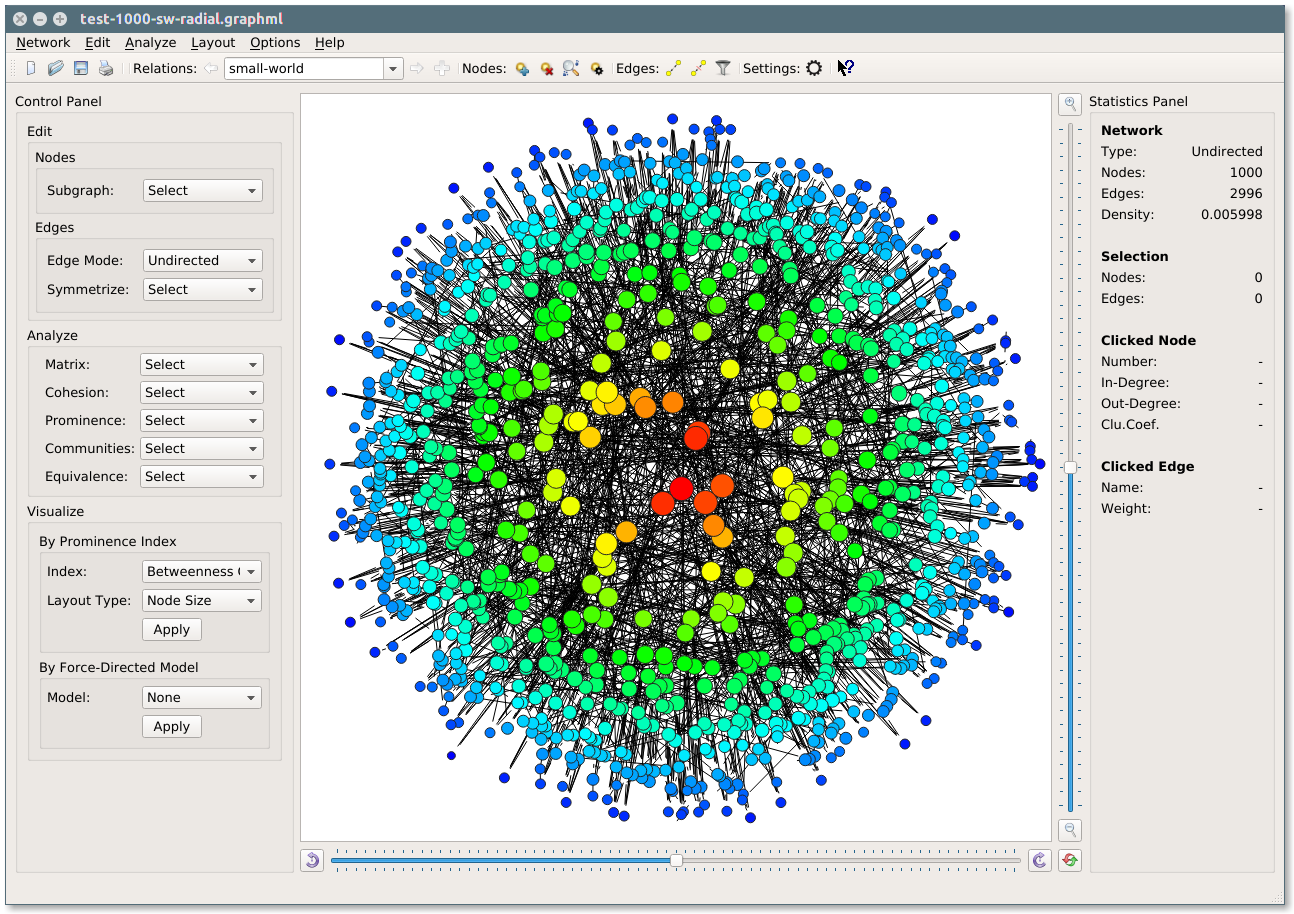Example: Small-world random network of 1000 actors created by SocNetV. Actor positions, colors and sizes reflect their betweenness centrality

#### Erdős–Rényi (ER) networks

The Erdős–Rényi networks are random networks created according by either of two related modes:

• the $$G(n, M)$$ model, introduced by Paul Erdős and Alfréd Rényi in 1959, where all networks of $$n$$ nodes and $$M$$ edges are equally likely.
• the $$G(n, p)$$ model, introduced by Edgar Gilbert also in 1959, where each edge has probability $$p$$ of being present or absent, independently of the other edges.

SocNetV generates Erdős–Rényi (ER) networks of both models.

In the $$G(n, M)$$ model, a graph of $$n$$ nodes and $$M$$ edges is created by randomly selecting two actors and adding a link between them until $$M$$ edges have been created. In the $$G(n, p)$$ model, for every possible pair of actors an edge is added with a Bernoulli trial, given the user-defined possibility $$p$$.

From the menu Network select Create Random Network > Erdos-Renyi.

In the dialog, enter the desired number of nodes $$n$$ and select a model. According to model selection you should either enter the edge probability $$p$$ or the number of edges $$M$$ in the final network.

You may also select if the final network will be undirected or directed and if you want to allow nodes to link to themselves (diagonal non zero).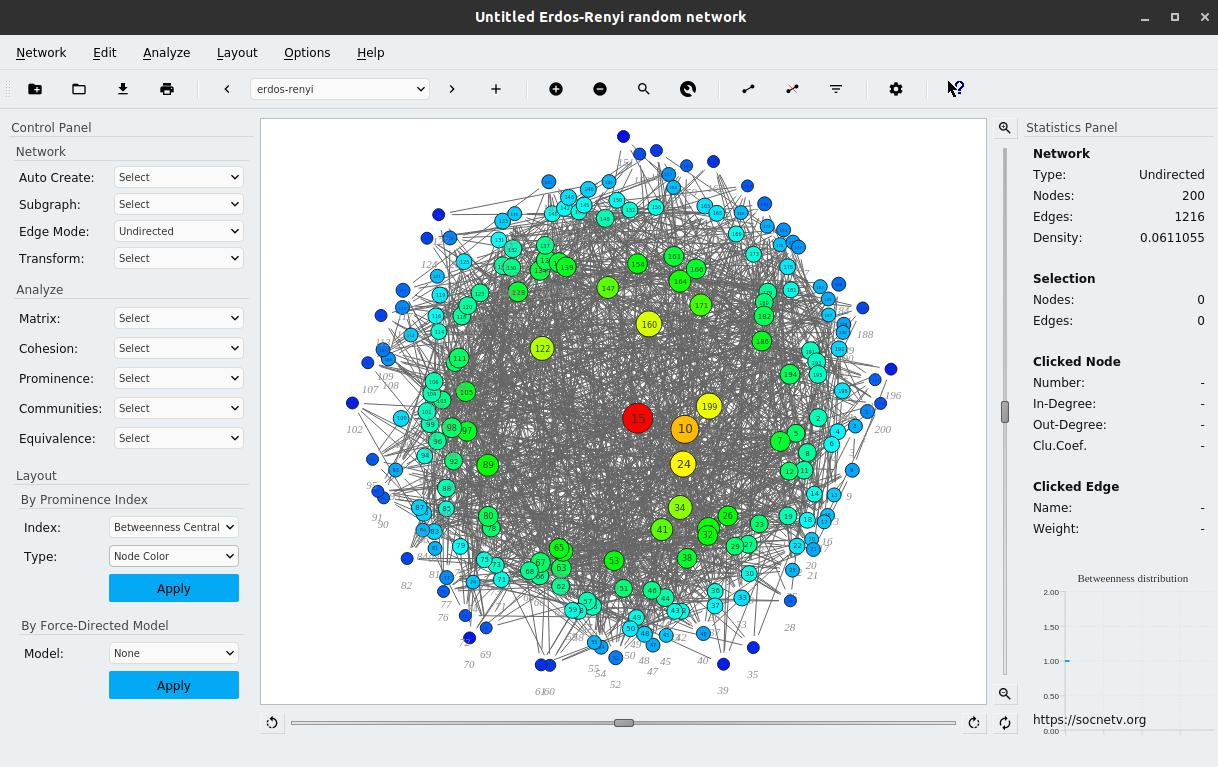Erdos-Renyi random network

#### Lattice networks

SocNetV can create regular lattices of arbitrary dimensions.

You can define the lattice dimension d, and the length/size l of each dimension.

Also, you can define custom neighborhood size n, which is the distance within which the neighbors on the lattice will be connected.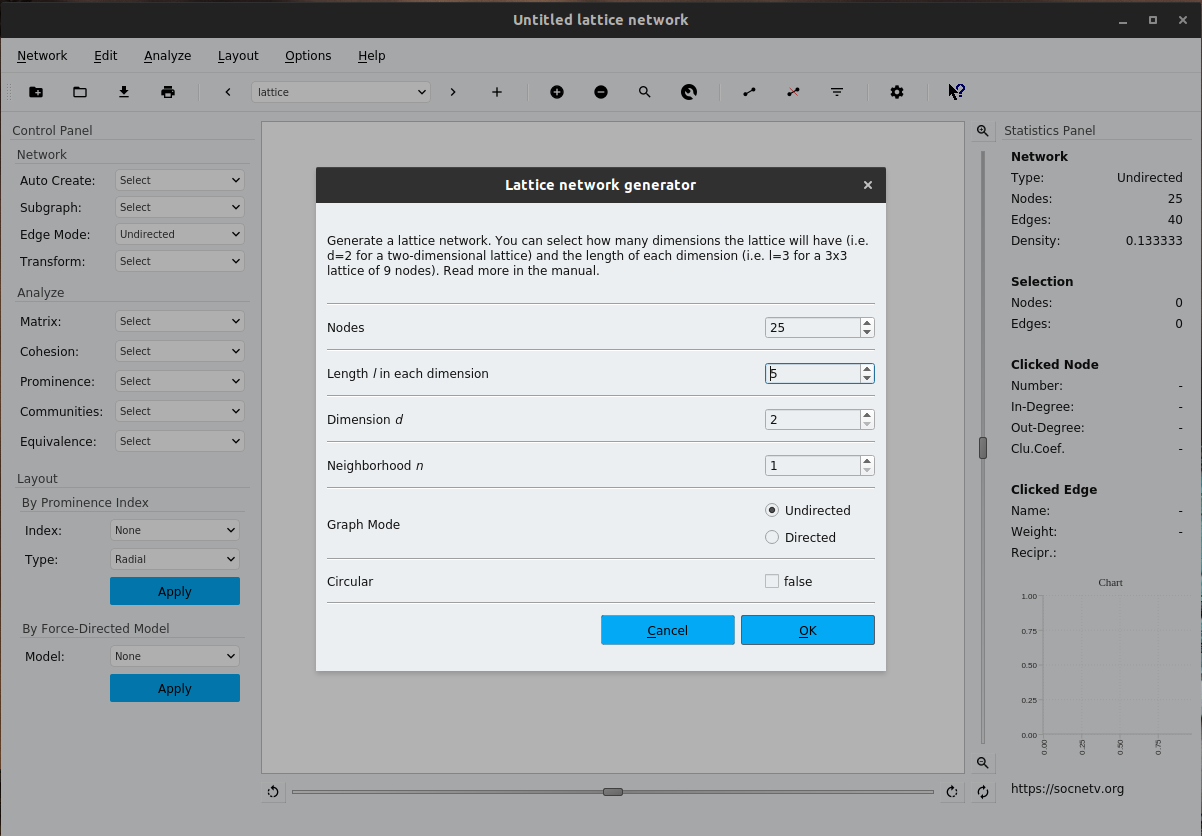lattice generator

#### d-regular networks

A d-regular network is that in which each actors has the same number of "neighbours" or the same degree d. Nodes are arbitrarily linked with each other other.

#### Ring lattices

Ring lattices (or physicist's lattices) are 'random' networks where all nodes are positioned in a ring. Each one has the same even degree (number of edges) d with her "neighbourhood", namely she is linked with the d/2 nodes before and d/2 nodes after her. For instance in a 4-lattice of 10 nodes, node 6 will be linked with 4, 5, 7 and 8. To create a ring lattice network click Network > Create Random Network > Ring Lattice (or press Shift+L). You will be asked for the number of nodes and the degree of each node.

## Networks of webpages using the Web Crawler

SocNetV includes a simple web crawler, which consists of two parts: a spider and a parser.

The spider visits a given initial URL (i.e. a website or a webpage) and downloads its HTML code.

The parser scans the code for 'href' links to other pages (internal or external) and adds them to a queue of URLs (called frontier).

As URLs are added in the queue, the spider visits them and downloads their HTML which is scanned for more links by the parser, and so on... The end result is the 'network' of all visited webpages as nodes and their real links as edges.

To start the web crawler, go to menu Network > Web Crawler or press Shift+C.

A dialog will appear, where you enter the initial web page (URL).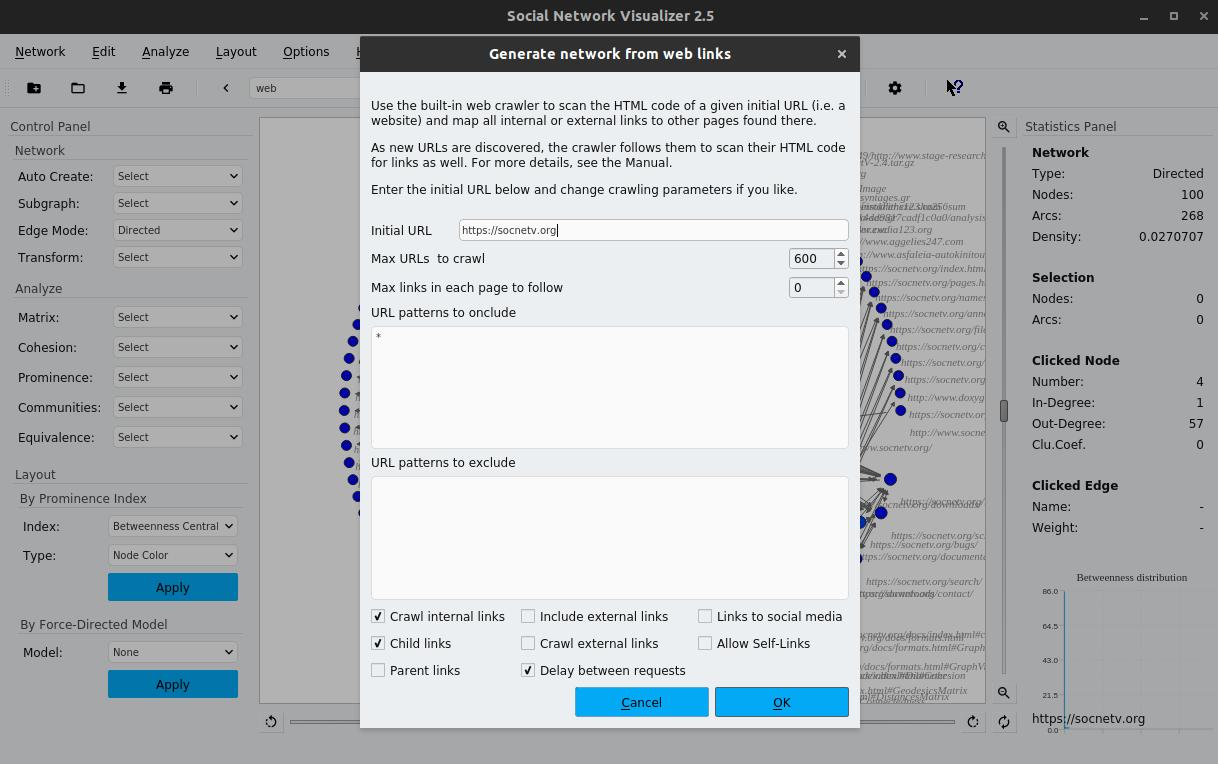Web crawler dialog

You may also limit the web crawler (after all you don't want to crawl the whole internet!):

Max URLs to crawl, this is the max nodes in the resulting network.

Max links per page, the amount of links in each page to consider.

Except the initial url and the limits, you can also specify patterns of urls to include or exclude, types of links to follow (internal, external or both) as well as if you want delay between requests (strongly advised)

Once you're ready, press OK and the crawler will start its job!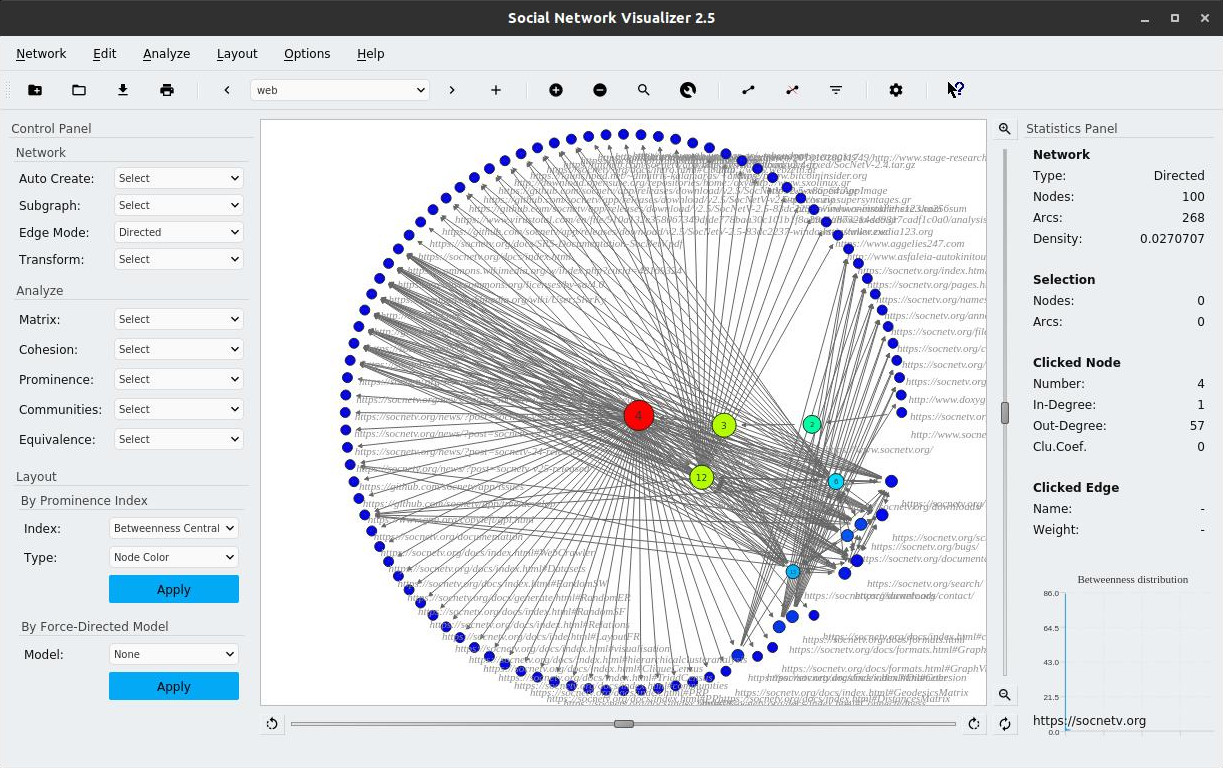Web crawler dialog
Note
By default the spider will crawl only internal links and use delay between network calls.
Warning
The parser searches for 'href' links only in the body section of the HTML code.

# Analysis methods

Once you load or create a network in SocNetV, you may use the options in the Analyze menu to compute graph and social network analysis measures.

## Matrix Analysis

Options to compute, display and analyze various matrices based on the adjacency matrix of the current network.

Available options:

Displays the adjacency matrix A of the active network. The matrix is displayed in HTML format in your default web browser.

The adjacency matrix of a social network is a matrix where each element a(i,j) is equal to the weight of the arc from actor (node) i to actor j.

If the actors are not connected, then a(i,j)=0.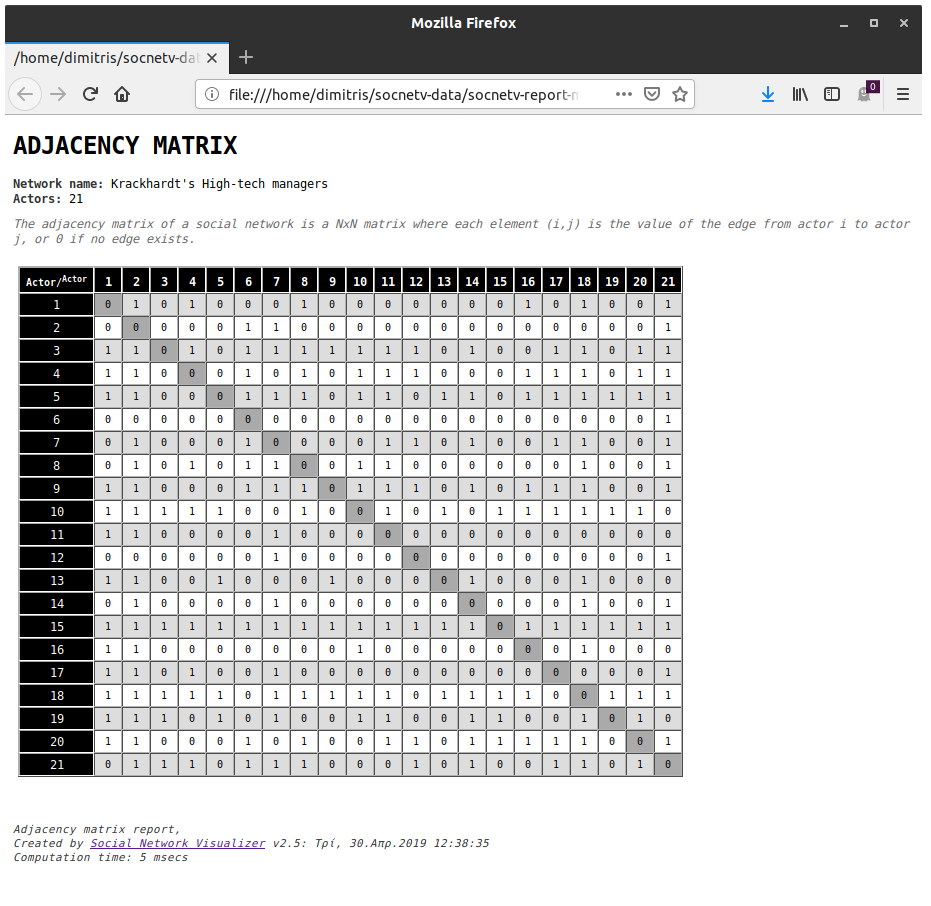Example: Adjacency matrix of Krackhardt High tech managers network

This option computes the inverse A-1 of the adjacency matrix.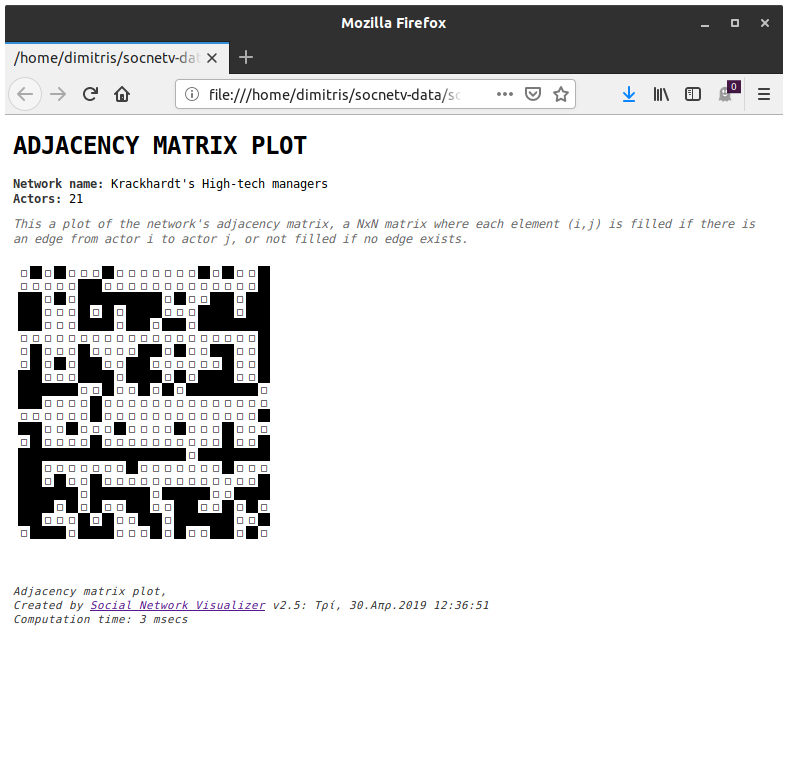Example: Adjacency matrix plot of a network

This option computes the transpose of the adjacency matrix.

#### CocitationMatrix

Computes the Cocitation matrix, C = AT * A.

C is a nxn symmetric matrix where each element (i,j) is the number of actors that have outbound ties/links to both actors i and j.

The diagonal elements, Cii, of the Cocitation matrix are equal to the number of inbound edges of i (inDegree).

#### Degree Matrix

This option computes the Degree matrix of the network. The Degree Matrix is diagonal matrix which contains information about the degree of each graph vertex (row of the adjacency matrix)

#### Laplacian Matrix

This option computes the Laplacian matrix of the network. The Laplacian is a NxN matrix L = D - A where D is the degree matrix of A

## Cohesion measures

The next option in the Analysis menu focus on basic network/graph measures, such as the geodesic distance between nodes, the mean distance between all nodes, the diameter of the graph, the number of geodesics between nodes and the eccentricity of each node. Each option is explained below.

#### Reciprocity

Reciprocity, r, is a measure of the likelihood of vertices in a directed network to be mutually linked.

SocNetV supports two different methods to index the degree of reciprocity in a social network: The arc reciprocity, which is the fraction of reciprocated ties over all actual ties in the network. The dyad reciprocity which is the fraction of actor pairs that have reciprocated ties over all pairs of actors that have any connection.

In a directed network, the arc reciprocity measures the proportion of directed edges that are bidirectional. If the reciprocity is 1, then the adjacency matrix is structurally symmetric.

Likewise, in a directed network, the dyad reciprocity measures the proportion of connected actor dyads that have bidirectional ties between them.

In an undirected graph, all edges are reciprocal. Thus the reciprocity of the graph is always 1.

Reciprocity can be computed on undirected, directed, and weighted graphs.

#### Symmetry Test

The Symmetry Test reports whether the network is symmetric or not. A network is called "symmetric" if for every edge $$(i,j)$$ in the set E of the corresponding graph $$G(V,E)$$, the 'opposite' $$(j,i)$$ edge also exists in $$E$$. In other words, when the adjacency matrix is symmetric.

#### Distance

Computes the geodesic distance (length of shortest path) between two actors.

In graph theory, the shortest path between two vertices of the graph is called "geodesic".

Thus, the geodesic distance of two nodes in a social network is the length of the shortest path between the corresponding vertices in the graph G(V,E).

By clicking on the Analyze -> Cohesion -> Distance option (or pressing Ctrl+G, Ctrl+G) you will be asked for source and target nodes. Then their distance will be calculated and displayed.

#### Average Distance

The average or mean distance in a social network is the average length of all shortest paths (geodesics) between all pairs of connected vertices in the corresponding graph.

In undirected or strongly connected directed networks, the formula used to compute Average Graph Distance is:

$\bar {d} = \frac { \sum_{u \ne v } { \{ d(u,v), \forall u,v \in V \} } } { n \cdot (n-1) }$

where the denominator is the sum of all pairs of vertices.

It can be proved that in connected networks of n actors, the least upper bound of the Average Distance is $$n+1 / 3$$ (Doyle & Graver, 1977).

In the case of disconnected networks/graphs, the denominator used is the total number of existing paths between connected vertices.

In graph theory, the Average Distance is considered to be a natural measure of the compactness of a graph.

#### Distances Matrix

The Analyze -> Cohesion -> Distances Matrix menu option displays the matrix of geodesic distances between all pairs of nodes in the social network.

A distances matrix is a $$N * N$$ square matrix, in which the $$(i,j)$$ element is the distance from node i to node j.

#### Geodesics Matrix

This option displays a n x n square matrix, where the $$(i,j)$$ element is the number of geodesics between node i and node j. The produced matrix, called sigma matrix, is used in Centralities calculation (see below).

#### Eccentricity

The eccentricity or association number of each node $$u$$ is the largest geodesic distance between that node and every other node in the network.

$\epsilon_u = \max { \{ d(u,v), \forall v \in V \} }$

Therefore, the measure reflects how far, at most, is each node from every other node.

The Eccentricity can be calculated in both graphs and digraphs but is usually best suited for undirected graphs.

It can also be calculated in weighted graphs although the weight of each edge (v,u) in E is always considered to be 1.

#### Diameter

The diameter of a social network is the maximum eccentricity of any vertex in the corresponding graph G(V,E), that is the maximum distance between any two connected nodes.

$D = \max { \{ d(u,v), \forall u,v \in V \} }$

#### Connectedness

Checks whether the network is a connected graph, a weakly connected digraph or a disconnected graph/digraph.

In graph theory, a graph is connected if there is a path between every pair of nodes.

A digraph is strongly connected if there the a path from i to j and from j to i for all pair of nodes $$(i,j)$$.

A digraph is weakly connected if at least a pair of nodes are joined by a semipath.

A digraph or a graph is disconnected if at least one node is isolate.

#### Walks of a given length

Clicking this option asks for a desired walk length (max: n-1). Then SocNetV calculates and displays a square matrix where each element $$(i,j)$$ is the number of walks of the given length between the corresponding pair of nodes i and j.

A walk is a sequence of alternating vertices and edges such as

v0e1, v1e2, v2e3, …, ekvk, where each edge, ei is defined as ei = {vi-1, vi}.

This function calculates the number of walks of the given length between each pair of nodes, by studying the powers of the sociomatrix.

#### Total Walks

Calculates and displays a (n x n) square matrix whose elements denote the number of walks of any length between each pair of nodes. The algorithm is based on the powers of the sociomatrix.

Warning
This function is VERY SLOW on large networks (n > 50), since it will calculate all powers of the sociomatrix up to (n-1) in order to find out all possible walks. If you need to make a simple reachability test, we advise to use the Reachability Matrix function instead.

#### Reachability Matrix

Calculates the reachability matrix XR of the graph where each $$(i,j)$$ element is 1 if nodes i and j are reachable, otherwise is 0.

This function is based on the Distances Matrix; it checks whether the corresponding element of the Distances matrix is not zero. If it is not zero, then the nodes $$(i,j)$$ are reachable and the XR element is 1.

#### Clustering Coefficient

In graph theory, a clustering coefficient reflects the degree to which the nodes tend to cluster together. In social network analysis, it is often used to characterize the transitivity of a network.

There are two versions of Clustering Coefficient: the global and the local.

The global Clustering Coefficient (often called transitivity, see Wasserman and Faust, 1994, page 243) is based on triplets of nodes to give an indication of the overall clustering in the whole network.

A triplet consists of three connected nodes. A triangle therefore includes three closed triplets, one centered on each of the nodes.

The global clustering coefficient is the number of closed triplets (or 3 x triangles) over the total number of triplets (both open and closed). This metric can be applied to both undirected and directed networks.

The local Clustering Coefficient (introduced by Duncan J. Watts and Steven Strogatz in 1998) is an indication of the embeddedness of single nodes, and it is also used as an indication of the network transitivity.

Specifically, the Clustering Coefficient $$C$$ of a node quantifies how close the node and its neighbors are to being a complete subgraph (clique).

Let $$k_{i}$$ the number of vertices, $$|N_{i}$$, in the neighbourhood, $$N_{i}$$, of a node $$i$$. In a directed network, the clustering coefficient is computed with the formula:

$C_i = \frac{|\{e_{jk}: v_j,v_k \in N_i, e_{jk} \in E\}|}{k_i(k_i-1)}$

In undirected networks, the formula is:

$C_i = \frac{2|\{e_{jk}: v_j,v_k \in N_i, e_{jk} \in E\}|}{k_i(k_i-1)}.$

If the network relation represents friendships among actors, the clustering coefficient $$C_i$$ of an actor i, measures the ratio of existing friendships between any two of that actor's friends relative to all possible friendships between her friends (the situation where the subgraph is complete).

A value close to one indicates that the node is involved in many transitive relations.

SocNetV also computes the network average clustering coefficient (Watts and Strogatz):

$\bar{C} = \frac{1}{n}\sum_{i=1}^{n} C_i$

Note
The clustering can be used to determine whether a network is a small-world or not.

For the ring lattice the clustering coeffient is

$C(0)=\frac{3(K-2)}{4(K-1)}$

tending to $$3/4$$ as $$K$$ grows, where K the mean degree.

Tip: All the basic network statistics, such as nodes, edges and density are displayed and automatically updated in the Analysis tab of the left dock in SocNetV main window.

## Centralities and Prestige

The last option in the Analysis menu opens the "Centrality and Prestige" sub-menu.

Social network analysts use various measures or (indices) to calculate how 'prominent' or important each actor (node) is inside a network (graph). For instance, we might want to know how important is a person inside her friendship network or how critical is a power station inside the power company grid...

Although there are various metrics, focusing on different graph notions and applying to different graph types, they are usually refered to as 'centralities' collectively.

SocNetV follows the conceptualization of prominence that Wasserman and Faust as well as Knoke and Burt use in their essays. To our understanding, all indices attempt to measure the visibility, the importance or the "prominence" of each node. But we distinguish two types of prominence: Centrality and Prestige.

Centrality measures attempt to quantify how much central is each actor inside the social network. To do so, these measures usually examine the ties attached to an actor as well as the geodesic distances (shortest path lengths) to other actors.

Most Centrality indices were designed for undirected graphs (symmetric), where the relations are non-directional. For instance, SocNetV can compute BC Betweenness, CC Closeness, IRCC Influence Range Closeness Centrality, DC Degree, SC Stress, EC Eccentricity and IC Information centrality indices.

For digraphs, where the relations are directional, most centrality measures can also be calculated by focusing on "choices made" (or outEdges).

But due to the nature of the directional relations in digraphs, the social networks researcher usually needs to measure the "prestige" (as known as status, rank or popularity) of each node. This can be done with Prestige measures, which focus on "choices received" (or nominations) of each node rather than "choices made" by that node. Given that Prestige measures are based on the inbound ties to each node from all others, these indices can only be calculated on directed graphs.

Centrality measures are calculated for each node (node Centrality) and for the whole network (group Centrality). Thus, when you click on a centrality option, SocNetV will calculate the corresponding index of every node and the whole network and it will display them in a new window (a small text editor). From there you can save the analysis into a text file of your choice. By default, analysis files are saved on bin/ subfolder.

#### Degree Centrality (DC)

The DC measure quantifies how many ties a node has to other nodes in the network. In social network theory, this index is often considered a measure of actor activity. It can be computed in both undirected and directed networks/relations, but is usually best suited for undirected ones.

Mathematically, in undirected graphs, the DC index of each vertex $$u$$ is the number of edges attached to it.

In directed graphs, the DC is the total number of arcs (outEdges) starting from $$u$$ (outDegree).

The index can be computed in weighted graphs as well. In that case, the DC of each node $$u$$ is the sum of weights of all edges/outEdges attached to $$u$$.

Along with other metrics which are based on the notion of distance (closeness, eccentricity etc), the DC falls in the category of reachability measures.

To compute Degree Centralization (or Group Degree Centrality), SocNetV uses the Freeman's formula for unvalued graphs.

$GDC = \frac { \sum { ( maxDC' - DC' ) } } { (N-1) \cdot (N-2) / (2 \cdot N - 1) }$

Note
In valued (or weighted) graphs, GDC cannot be computed with Freeman's formula. As a measure of degree centralization, one can use DC variance or mean instead.

#### Closeness Centrality (CC)

This CC index focuses on how close each node is to all other nodes in the network.

Nodes with high Closeness Centrality are those who can reach many other nodes in few steps. The idea is that a node is more central if it can quickly interact with more of the others. CC is also interpreted as the ability to access information through the "grapevine" of network members.

For each node $$u$$, the CC score is the inverse sum of geodesic distances from that node to every other node.

$CC_u = \frac { 1 } { \sum_{v \in E} { d(u,v) } }$

This index can be calculated in graphs and strongly connected digraphs (that is, if there is a directed path from v to u for all nodes v and u in the graph). If there are isolate nodes in the network, they are dropped by default. In not strongly connected digraphs, the ordinary CC is undefined. In that case, you can use the Influence Range Closeness Centrality index.

CC can also be calculated in weighted graphs although the value of each edge (u,v) is always considered to be 1.

The maximum value of CC is 1/(N-1), when the node is adjacent to all others. Thus the standardized CC index (CC') is calculated by (N-1) * CC.

Group CC is calculated using Freeman's general formula, in undirected graphs:

$GCC = \frac { \sum { ( maxCC' - CC' ) } } { (N-1) \cdot (N-2) / (2 \cdot N - 1) }$

Note
As with all centrality measures in directed graphs, CC considers only outbound edges. If you want to analyze inbound edges, use a Prestige measure, i.e. Proximity Prestige.

#### Influence Range Closeness Centrality (IRCC)

For each node u, IRCC is the standardized inverse average distance between u and every other node reachable from it.

This improved CC index is optimized for graphs and directed graphs which are not strongly connected. Unlike the ordinary CC, which is the inverted sum of distances from node v to all others (thus undefined if a node is isolated or the digraph is not strongly connected), the IRCC considers only distances from node u to nodes in its influence range J (nodes reachable from u).

The IRCC formula used is the ratio of the fraction of nodes reachable by u (|J|/(n-1)) to the average distance of these nodes from u sum(d(u,j))/|J|

$\frac { \frac { |J| } { (n-1) } } { \frac { \sum d(u,j) } { |J| } }$

#### Betweenness Centrality (BC)

For each node u, BC is the ratio of all geodesics between pairs of nodes which run through u. It reflects how often that node lies on the geodesics between the other nodes of the network.

The BC score of each actor can be interpreted as a measure of potential control as it quantifies just how much that actor acts as an intermediary to others. An actor which lies between many others is assumed to have a higher likelihood of being able to control information flow in the network.

In essence, BC assumes that communication in a network occurs along the shortest possible path, the geodesic. It totally neglects the possibility of communication along non-geodesic paths between actors. If you need a centrality index which considers all possible paths, use the Information Centrality (IC).

Note that betweenness centrality assumes that all geodesics have equal weight or are equally likely to be chosen for the flow of information between any two nodes. This is reasonable only on "regular" networks where all nodes have similar degrees. On networks with significant degree variance you might want to try using IC instead.

Also, BC is very sensitive to network dynamics, i.e. it changes a lot when we add or remove a vertex or an edge.

This index can be calculated in both graphs and digraphs but is usually best suited for undirected graphs. It can also be calculated in weighted graphs although the weight of each edge (u,v) in E is always considered to be 1.

#### Stress Centrality (SC)

The SC of each node u is the total number of geodesics between all other nodes which run through u. When one node falls on all geodesics between all the remaining (N-1) nodes, then we have a star graph with maximum Stress Centrality. This index reflects how often a node lies on the geodesics between other nodes.

This index can be calculated in both graphs and digraphs but is usually best suited for undirected graphs. It can also be calculated in weighted graphs although the weight of each edge (v,u) in E is always considered to be 1

This index was introduced by Shimbel (1953).

#### Eccentricity Centrality (EC) or Harary Graph Centrality

For each node u, the Eccentricity Centrality is the inverse of the largest geodesic distance (u,v) to every other node v in the network. Therefore, the EC score reflects how close is each node to every other node and therefore to the middle of the network.

Nodes with high EC score have short distances to other nodes in the graph and therefore are likely to be near the middle of the network. Nodes with low EC score have longer distances to some other nodes in the graph, and therefore are most likely towards the "edge" of the network.

This index can be calculated in both graphs and digraphs but is usually best suited for undirected graphs. It can also be calculated in weighted graphs although the weight of each edge (v,u) in E is always considered to be 1.

The EC is also known as Graph Centrality (Hage and Harary, 1995).

#### Power Centrality (PC)

The Power Centrality (PC) is a a generalised degree centrality measure suggested by Gil and Schmidt.

For each node u, this index sums its degree (with weight 1), with the size of the 2nd-order neighbourhood (with weight 2), and in general, with the size of the kth order neighbourhood (with weight k).

Thus, for each node u the most important other nodes are its immediate neighbours and then in decreasing importance the nodes of the 2nd-order neighbourhood, 3rd-order neighbourhood etc. For each node, the sum obtained is normalised by the total numbers of nodes in the same component minus 1.

This index can be calculated in both graphs and digraphs but is usually best suited for undirected graphs. It can also be calculated in weighted graphs although the weight of each edge (u,v) in E is always considered to be 1 (therefore not considered).

#### Information Centrality (IC)

The Information Centrality (IC) is an index suggested by Stephenson and Zelen (1989) which focuses on how information might flow through many different paths. Unlike SC and BC, the IC metric uses all paths between actors weighted by strength of tie and distance.

The IC' score is the standardized IC (IC divided by the sumIC) and can be seen as the proportion of total information flow that is controlled by each actor.

Note that standard IC' values sum to unity, unlike most other centrality measures.

Since there is no known generalization of Stephenson & Zelen's theory for information centrality to directional relations, the index should be calculated only for undirected graphs and is more meaningful in weighted graphs/networks.

Note: To compute this index, SocNetV drops all isolated nodes and symmetrizes (if needed) the adjacency matrix even when the graph is directed (Wasserman & Faust, p. 196).

ALGORITHM: In order to calculate the IC index of each actor, we create a N x N matrix A from the (symmetrized) sociomatrix with:

$A_ii = 1 + d_i$

$A_ij = 1 \space if \space (i,j)=0$

$A_ij = 1 -w_{ij} \space if \space (i,j) = w_{ij}$

Next, we compute the inverse matrix of A, for instance C, using LU decomposition. Note that we can always compute C since the matrix A is always a diagonally strong matrix, hence it is always invertible.

Finally, IC is computed by the formula:

$\frac { IC_i - 1 } { C_{ii} + \frac { T-2 \cdot R } { N } }$

where: T is the trace of matrix C (the sum of diagonal elements) and R is the sum of the elements of any row (since all rows of C have the same sum)

IC has a minimum value but not a maximum.

#### Eigenvector Centrality or Gould index

Computes the Eigenvector centrality of each node in a social network which is defined as the ith element of the leading eigenvector of the adjacency matrix. The leading eigenvector is the eigenvector corresponding to the largest positive eigenvalue.

The Eigenvector Centrality, proposed by Bonacich (1989), is an extension of the simpler Degree Centrality because it gives each actor a score proportional to the scores of its neighbors.

Thus, a node may be important, in terms of its EC, because it has lots of ties or it has fewer ties to important other nodes

#### Degree Prestige (DP) or InDegree Centrality

For each node u, this metric counts the number of inbound arcs at u. The index is meaningful in directed graphs as a measure of the prestige of each node. Thus it is called Degree Prestige (it is also known as InDegree Centrality). Note that in undirected graphs, the DP index is identical to Degree Centrality.

Actors with higher DP are considered more prominent among others because they receive more nominations or choices (they have larger inDegree). The largest the index is, the more prestigious is the node/actor.

This index can be calculated only for unvalued or valued digraphs. In weighted relations, DP is the sum of weights of all arcs/inLinks ending at node v.
In unvalued graphs, SocNetV computes Group Degree Prestige using the Freeman's formula .

Note: For valued or weighted graphs, we cannot calculate Group DC using Freeman's formula. You can use DP variance or mean instead.

#### PageRank prestige (PRP)

The PageRank prestige is an importance ranking for each node based on the structure of its incoming links/edges and the rank of the nodes linking to it.

The original PageRank algorithm, developed by Page and Brin (1997), focuses on how nodes are connected to each other, treating each link from a node as a citation/backlink/vote to another.

In essence, for each node the algorithm counts all incoming links (edges) to it, but it does so by not counting all links equally while it normalizes each in-link from a node by the total number of its outgoing edges.

The PR index for each node $$u$$ is computed iteratively by the formula:

$PR_u = \frac { 1- d } { N } + d \cdot \sum_{v \in M_u} \frac {PR_v }{DC_v}$

where $$u$$ is the node, $$d$$ is a dumping factor ( $$d = 0.85$$), $$N$$ the number of of vertices in the network $$M_u$$ all nodes which link to $$u$$ $$DC_v$$ the outDegree of node $$v$$

The PR values correspond to the principal eigenvector of the normalized link matrix.

This index can be calculated in both graphs and digraphs but is usually best suited for directed graphs since it is a prestige measure.

The PageRank prestige index can also be calculated in weighted graphs.

Note: In weighted relations, each backlink to a node u from another node v is considered to have weight=1 but it is normalized by the sum of outEdges weights (outDegree) of v. Therefore, nodes with high outLink weights give smaller percentage of their PR to node u.

#### Proximity Prestige (PP)

The Proximity Prestige index measures how proximate a node u is to the nodes in its influence domain $$I$$ - the influence domain I of a node is the number of other nodes that can reach it. Apparently, in this metric the proximity of each node u is based on distances to rather than distances from it. To put it simply, in PP what matters is how close are all the other nodes to node u.

The algorithm takes the average distance to node $$u$$ of all nodes in its influence domain, standardizes it by multiplying with $$(N-1)/|I|$$ and takes its reciprocal.

In essence, the formula SocNetV uses to calculate PP for every node $$u$$ is the ratio of the fraction of nodes that can reach node $$u$$, to the average distance of that nodes to $$u$$ (Wasserman & Faust, formula 5.25, p. 204):

$PP = \frac { \frac { I } { N-1 } } { \sum{d(v,u)} / I }$

where the sum is over every node $$v$$ in $$I$$.

## Community detection

#### Clique Census

The concept of a clique in every life is pretty simple: a clique is a group of people who interact with each other much more regularly and intensely than with other people not belonging in the clique. That is, a group of people form a clique if they are all connected to each other.

In formal mathematics, a clique C is any subset of vertices of an undirected graph G, such that its induced subgraph is complete. This means that every two distinct vertices in a clique are always adjacent.

In Social Network Analysis, the definition of a clique is much more narrow and precise:

A clique is the largest subgroup of actors in the social network who are all directly connected to each other. In terms of graph theory, this notion is the same as a maximal complete subgraph of the equivalent graph of the social network. The word maximal means that for each clique the group of its members is expanded to include as many actors as possible; no other actors can be added to the clique. Essentially, a clique in Social Network Analysis consists of several overlapping closed triads.

SocNetV applies the Bron–Kerbosch algorithm to find all maximal cliques in an undirected or directed graph. It produces a census of all MAXIMAL cliques in the network and reports some useful statistics about these. The clique census report includes disaggregation by vertex and co-membership information.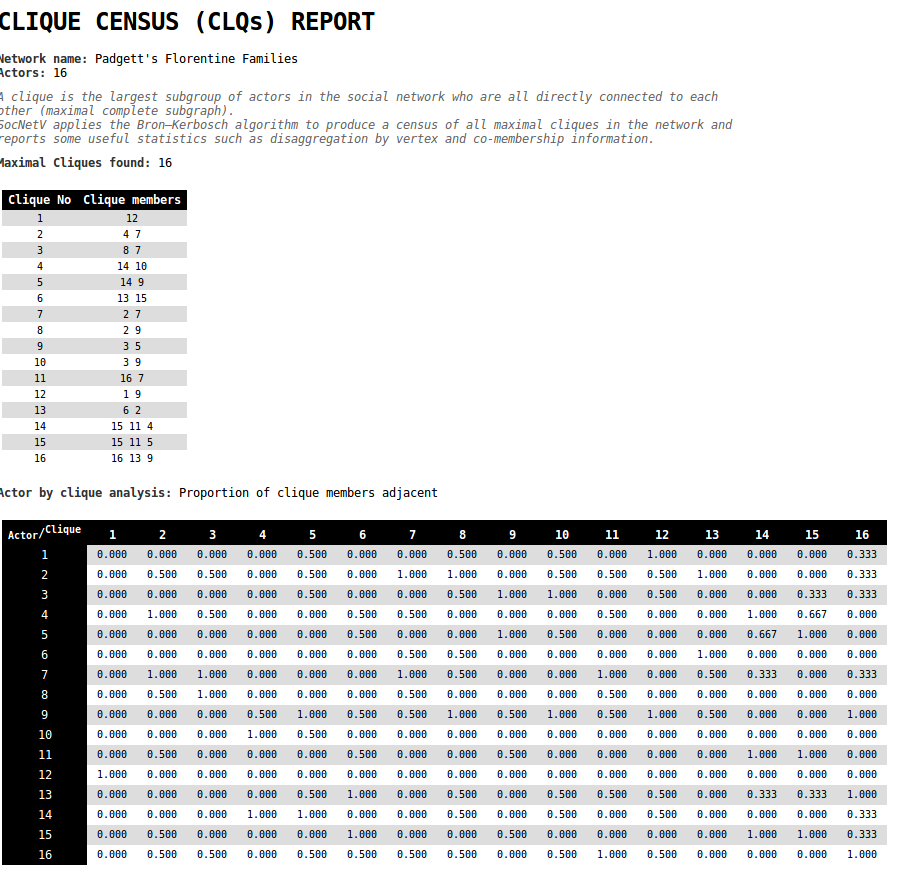Clique census of Florentine families
Warning
This computation can be slow in very large / dense social networks! In general, the clique problem (the problem of finding maximal cliques in a given graph) is NP-complete.

By clicking the "Triad Census" menu option, SocNetV will examine each of the triads present in the current network, and count how many of these belong to a certain triad type.

Some background:

In any network of N actors, there are C(N,3) triads. For instance, in a network of 6 actors there are C(4,3)=20 triads, whereas in a network of 10 actors there are C(10,3)=60 triads.

In any case, though, there can be only sixteen different triad types (isomophism classes).

Every one of the C(N,3) triads of a network must belong (be isomorphic) to one of these sixteen types.

A Triad Census is a method which counts all the different types (classes) of observed triads within a network.

The triad types are coded and labeled according to their number of mutual, asymmetric and non-existent (null) dyads.

SocNetV follows the M-A-N labeling scheme, as described by Holland, Leinhardt and Davis in their studies. In the M-A-N scheme, each triad type has a label with four characters:

• The first character is the number of mutual (M) duads in the triad. Possible values: 0, 1, 2, 3.
• The second character is the number of asymmetric (A) duads in the triad. Possible values: 0, 1, 2, 3.
• The third character is the number of null (N) duads in the triad. Possible values: 0, 1, 2, 3.
• The fourth character is infered from features or the nature of the triad, i.e. presence of cycle or transitivity. Possible values: none, D ("Down"), U ("Up"), C ("Cyclic"), T ("Transitive")

In the seven rows below, you can see all the sixteen triad types (classes). Within each row, all the triad types have the same number of arcs present:

003
012
102     021D    021U    021C
111D    111U    030T    030C
201     120D    120U    120C
210
300


So, when you click on Triad Census menu option, SocNetV calculates and displays a vector T of length 16. Each vector element (Tu) is the frequency of each one triad type inside the active network, i.e. T003 = 3. Furthermore, the order of the elements of vector T is the same as the aforementioned ordering of the triad types:

T = [ T003, T012, T102, T021D, T021U, T021C, T111D, T111U, T030T, T030C, T201, T120D, T120U, T120C, T210, T300 ]

Apparently, the sum of all these frequencies Tu is C(N,3).

## Structural Equivalence methods

A key notion in SNA is that of structural equivalence. The idea is to map the relationships in a graph by creating classes or groups of actors who are equivalent in some sense.

One way to do that, to identify groups of actors who are structurally equivalent, is to examine the relationships between them for similarity patterns.

There are many methods to measure the similarity or dissimilarity of actors in a network.

SocNetV supports the following methods:

• Similarity by measure
• Pearson Correlation Coefficients
• Tie profile dissimilarities

By applying one of these methods, SocNetV creates a pair-wise actor similarity/dissimilarity matrix.

#### Actor Similarity (by measure)

Computes a pair-wise actor similarity matrix, where each element (i,j) is the ratio of tie (or distance) matches of actors i and j to all other actors.

SocNetV supports the following measures:

• Simple Matching (Exact Matches): Proportion of tie/distance matches (present or absent) between two actors
• Jaccard Index (Positive Matches or Co-citation): Percentage of same ties/distances reported by both actors to the total number of ties reported.
• Hamming distance: Number of ties/distances which differ between each pair of actors.
• Cosine similarity
• Euclidean distance

For instance, in the case of Simple Matching, the similarity matrix depicts the ratios of exact matches of pairs of actors to all other actors. If the element (i,j) = 0.5, this means that actors i and j have the same ties present or absent to other actors 50% of the time.

These measures of similarity are particularly useful when ties are binary (not valued).

Note that in very sparse networks (very low density), measures such as exact matches, correlation and distance will show little variation among the actors, causing difficulty in classifying the actors in structural equivalence classes.

#### Pearson Correlation Coefficients

Computes a correlation matrix, where the elements are the Pearson correlation coefficients between pairs of actors in terms of their tie profiles or distances (in, out or both).

The Pearson product-moment correlation coefficient (PPMCC or PCC or Pearson's r) is a measure of the linear dependence/association between two variables X and Y.

This correlation measure of similarity is particularly useful when ties are valued/weighted denoting strength, cost or probability.

#### Tie Profile Dissimilarities

Computes a matrix of tie profile distances/dissimilarities between all pairs of actors/nodes in the social network using an ordinary metric such as Euclidean distance, Manhattan distance, Jaccard distance or Hamming distance).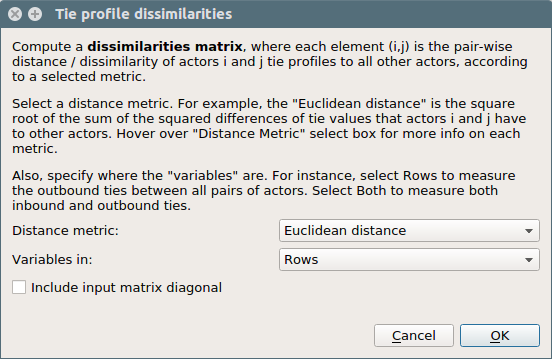Dissimilarities dialog example

The resulted distance matrix is a n x n matrix, in which the (i,j) element is the distance or dissimilarity between the tie profiles of node i and node j.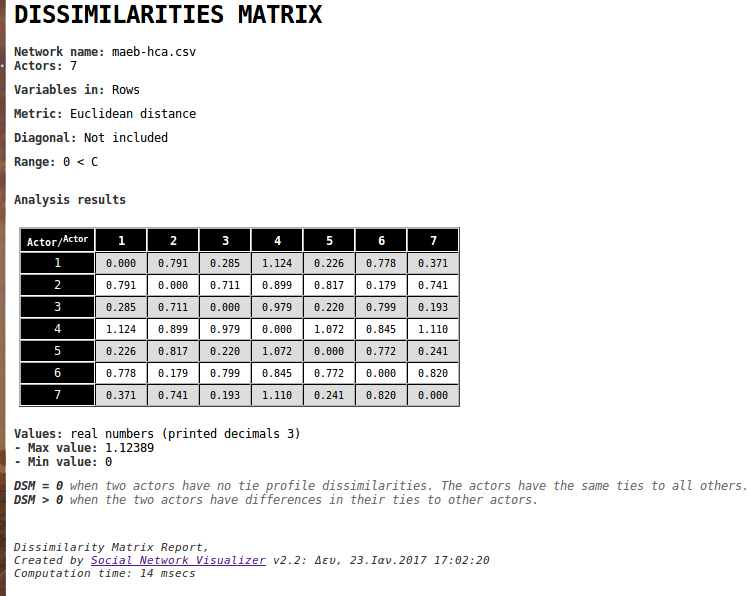Example of tie profile disssimilarities report

#### Hierarchical cluster analysis (HCA)

Hierarchical clustering (or hierarchical cluster analysis, HCA) is a method of cluster analysis which builds a hierarchy of clusters, based on their elements dissimilarity. In SNA context these clusters usually consist of network actors.

This method takes the social network distance matrix as input and uses the Agglomerative "bottom up" approach where each actor starts in its own cluster (Level 0). In each subsequent Level, as we move up the clustering hierarchy, a pair of clusters are merged into a larger cluster, until all actors end up in the same cluster.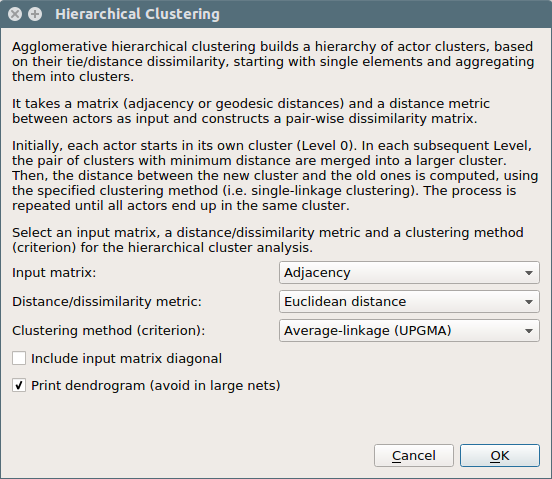HCA dialog where you select input matrix, dissimilarity metric and linkage criterion/method

To decide which clusters should be combined at each level, a measure of dissimilarity between sets of observations is required. This measure consists of a metric for the distance between actors i.e. manhattan distance) and a linkage criterion (i.e. single-linkage clustering).

This linkage criterion (essentially a definition of distance between clusters), differentiates between the different HCA methods.

The result of Hierarchical Cluster Analysis is the clusters per level and a dendrogram:Example dendrogram of HCA
Note
Note that the complexity of agglomerative clustering is O( n^2 log(n) ), therefore is too slow for large data sets.

# Visualization and layout algorithms

SocNetV supports two kinds of network visualizations: By Prominence index and Force-Directed, each one with various layout algorithms.

You can select visualizations from the menu "Layout" or by clicking on the checkboxes on the left dock.

## Prominence-based placement

SocNetV implements algorithms to layout the network so that each node takes a position that reflects its centrality or prestige status inside the network.

These algorithms supports all prominence (Centrality and Prestige) measures and a variety of layout types (circular, level, nodal size and color). In the left dock choose a prominence index, a layout type and then press the Apply button.

Please note that these algorithms do not take care of crossing links. They are only meaningful as a visual representation of the status of each node.

#### Circular

If you select circular layout type, all nodes will be repositioned on the circumferences of concentric circles of different radiuses.

Nodes with higher centrality or prestige are positioned closer to the centre of the screen.

#### On Levels

If you select the "on levels" layout type, all nodes will be repositioned on levels of different height.

More central nodes will appear towards the top of the screen.

#### Nodal size

In this layout type, the size of each node will change to reflect its centrality or prestige score. You can apply this layout type along with circular or level layout for a more meaningful visualization.

## Force-directed placement

In this model, the network is considered to be a dynamic system where every two actors are 'particles' mutually connected by a 'spring'.

Each spring has a desirable length, which corresponds to their graph theoretic distance.

In this way, the optimal layout of the graph is the state with the minimum imbalance.

The degree of imbalance is formulated as the total spring energy:

the square summation of the differences between desirable  distances and real ones for all pairs of particles


Initially, the particles/actors are placed on the vertices of a regular n-polygo

#### Spring Embedder

The Spring Embedder model (Eades, 1984), part of the Force Directed Placement (FDP) family, embeds a mechanical system in the graph by replacing nodes with rings and edges with springs which excert forces. In the words of Eades himself:

"The basic idea is as follows. To embed [lay out] a graph we replace the vertices by steel rings and replace each edge with a spring to form a mechanical system . . . The vertices are placed in some initial layout and let go so that the spring forces on the rings move the system to a minimal energy state."

In our implementation, every node $$u \in V$$ of the network is regarded as a physical body (i.e. ring) which exert repelling force $$F_{r}$$ to every other node. At the same time, every edge $$(u,v) \in E$$ becomes a "spring" which excert an attractive force $$F_{a}$$ between the nodes $$u$$ and $$v$$ it connects.

Following Eades, the forces of springs do now follow Hooke'w Law. Instead we assume weaker, logarithmic forces between edges $$(u,v) \in E$$:

$F_{a}^{u,v} = c_1 \cdot \log{ \frac{d_{u,v}}{c_2} }$

where $$d$$ the euclidean distance, the constant $$c_1 = 2$$ and the other constant $$c_2$$ is the "natural length" of the spring which is a function of screen/viewport area and the width of the vertex:

$$c_2 = vertexWidth + \sqrt{ \frac { screenArea } { |V| } }$$

The repelling force $$F_{r}$$ between every pair of nodes is computed with the formula:

$F_{r} = \frac { c_3 } { d_{u,v} ^2 }$

where $$c_3=1$$

These forces are applied to the nodes iteratively, pulling them closer together or pushing them further apart, until the system comes to an equilibrium state (node positions do not change anymore).

Note: Repulsive forces are calculated between every pair of vertices, but attractive forces are calculated only between neighbours (nodes connected by an edge/arc).

#### Fruchterman-Reingold

Fruchterman and Reingold (1991) refined the Spring Embedder model by replacing nodes with electrically charged or gravitational bodies.

In their words:

"Consider the following analogy: the vertices behave as atomic particles or celestial bodies, exerting attractive and repulsive forces on one another; the forces induce movement. Our algorithm will resemble molecular or planetary simulations, some- times called n -body problems. Following Eades, however, we know that we need not have a faithful simulation; we can apply unrealistic forces in an unrealistic manner. So, like Eades, we make only vertices that are neighbours attract each other, but all vertices repel each other. This is consistent with the asymmetry of our two guidelines above."

Again, only neighbouring vertices attract each other while all vertices repel each other.

In our implementation the attracting and repulsive forces are computed as follows:

AttractingForce = ( dist(v,u) * dist(v,u) ) / optimalDistance
RepulsiveForce = (optimalDistance * optimalDistance / dist(v,u))

where

optimalDistance= sqrt ( screenArea / |Vertices| )

Following Fruchterman and Reingold, for every vertex v we compute repulsive forces only for vertices within a circular area of radius 2*optimalDistance from v.